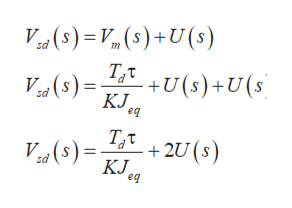# Tetwo-uagiee ur iCCaonITCompelsauor with sUL pomt wCigntng Is.r()y(t)sp[6.4.1]dsd V(s)u+'eq

Question
1 views
1. from the given equation 6.4.1, Determine the closed-loop disturbance to position transfer function, , assuming zero reference input, in terms of Jeq, K, τ, kp, ki and kd.   Assume a simulated disturbance voltage, Vsd, where the voltage applied to the motor is: second screenshot
check_circle

Step 1

Rewrite the equation 6.4.1 and substitute 0 for r(t).

Step 2

Take the Laplace transform,

Step 3

Calculate the value of disturbance voltage after putt...help_outlineImage TranscriptioncloseИ() - И,(5) +U(5) Т,т +U(s) + U (s (s) КЛ. eq Т.t V (s) 2U (s) КЛ. еq Ко fullscreen

### Want to see the full answer?

See Solution

#### Want to see this answer and more?

Solutions are written by subject experts who are available 24/7. Questions are typically answered within 1 hour.*

See Solution
*Response times may vary by subject and question.
Tagged in

### Electrical Engineering